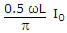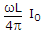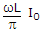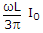# Electronics and Communication Engineering - Power Electronics

Exercise : Power Electronics - Section 6
26.
A single phase converter is fed by a source having an inductance L. If load current is I0, the decrease in output voltage due to overlap isExplanation:
No answer description is available. Let's discuss.

27.

Assertion (A): An ac regulator uses natural commutation

Reason (R): An ac regulator can be used for static on load tap changing of transformer.

Both A and R are correct and R is correct explanation of A
Both A and R correct but R is not correct explanation of A
A is correct but R is wrong
A is wrong but R is correct
Explanation:
No answer description is available. Let's discuss.

28.
When a UJT is used as a relaxation oscillator the current should be
less than Ip
more than Iv
more than Ip but less than Iv
negative
Explanation:
No answer description is available. Let's discuss.

29.
In auxiliary commutated chopper, the charging and discharging time of capacitor
are constant
may be constant or may depend on load current
none of the above